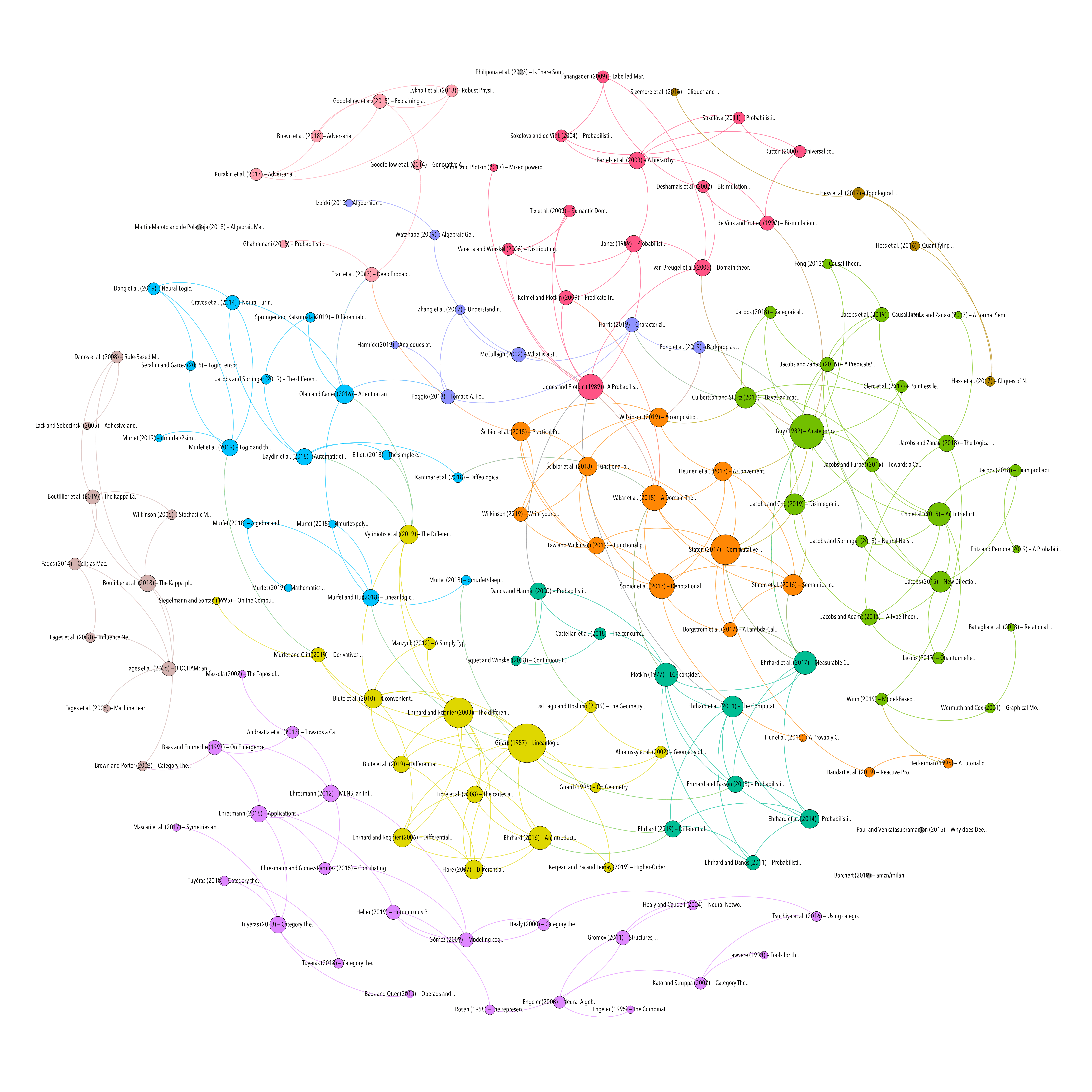MACHINE LEARNING

Derivatives of Turing machines in Linear Logic

Resource type
Authors/contributors
Title
Derivatives of Turing machines in Linear Logic
Abstract
We calculate denotations under the Sweedler semantics of the Ehrhard-Regnier derivatives of various encodings of Turing machines into linear logic. We show that these derivatives calculate the rate of change of probabilities naturally arising in the Sweedler semantics of linear logic proofs. The resulting theory is applied to the problem of synthesising Turing machines by gradient descent.
Publication
arXiv:1805.11813 [math]
Date
2019-01-28
Accessed
2019-11-21T20:33:27Z
Library Catalog
Extra
ZSCC: NoCitationData[s0] arXiv: 1805.11813
Notes
Comment: 62 pages, moved the section on naive Bayesian observers earlier (Section 6.2) with slight changes to notation, references added in the introduction to Section 7 and related work in Remark 7.16
Citation
Murfet, D., & Clift, J. (2019). Derivatives of Turing machines in Linear Logic. ArXiv:1805.11813 [Math]. Retrieved from http://arxiv.org/abs/1805.11813
CATEGORICAL LOGIC
DIFFERENTIAL CALCULUS
MACHINE LEARNING
MODEL CHECKING AND STATE MACHINES
Topic
Attachment
Processing time: 0.02 secondsGraph of references (from Zotero to Gephi via Zotnet with this script)